# Analytics Calculations: Math Functions

Moderator, Lightspeed Staff Posts: 81 moderator
edited October 2020
*Note, from this part of the Discussions, we will assume that you're comfortable with starting Calculations. Changing dimensions and measures, changing filters, and some of the basic starter calculations: addition, subtraction, division, multiplication, greater than, less than, equal to, single-criteria IF functions, and multiple criteria IF functions.

If you're in the early stages of your Analytics mastery, you may want to take a quick look at the related Discussions:

Multiplying by percentages in Analytics

Analytics provides lots of functions that perform different mathematical, logical, and table-based calculations. You can find a more in-depth catalogue of available functions on the Looker list of functions and operators.

To make it easier, we could categorize these in five different ways: Cell functionsDate functions, Logic Functions, Math functions, and Table functions

In this article, we'll be looking at ten of the Math based functions:
Large, Max, Mean/Median/Mode, Min, Round, Runningtotal, Small, Sum,

# Large

Returns the nth largest value from a range, where "n" is freely defined. Requires two arguments, the range, and which largest number.

For example, if I want to find my highest daily sales total, I could build...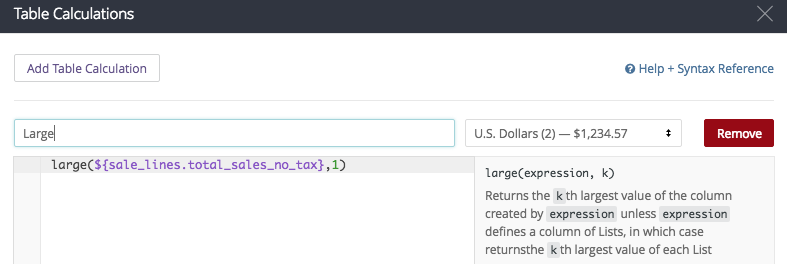My calculation is
large(\${sale_lines.total_sales_no_tax},1)

and if I have saved it properly...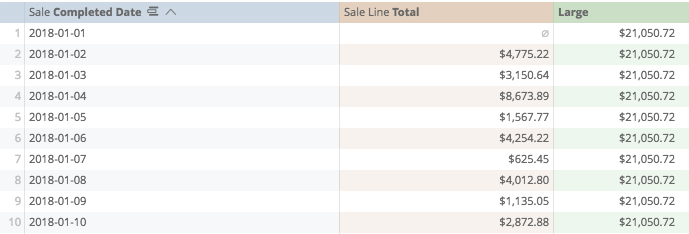Now, because I prepared my calculation to always look for the 1st largest value, I get the same value in every row. (in the above demonstration, \$21,050.72)

I could also perhaps return the row (from our logic calculations) and have it provide the highest on row 1, the second highest on row 2, the third highest on row 3, and so on.

In this instance, my calculation would look like:
large(\${sale_lines.total_sales_no_tax},(row()))

and when I save: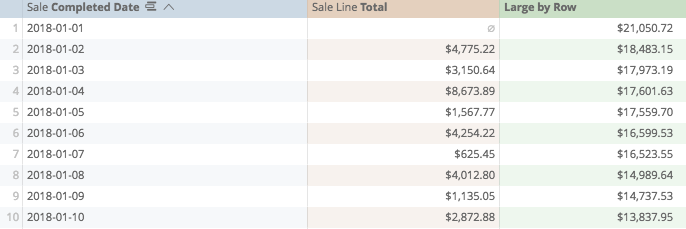# Max

Returns the largest value from a defined range. Required one argument: defined numeric range.

# Mean, Median, Mode

Returns the corresponding measure of average value from a range:
• Mean, corresponding to the mathematical average (sum of total/count of total),
• Median, corresponding to the central value of sorted results, eg, in 3 results, return the 2nd, in 5 results, return the 3rd, in 101 results, return the 51st (or the average of the two central values in an even number of results).
• Mode being the most common of results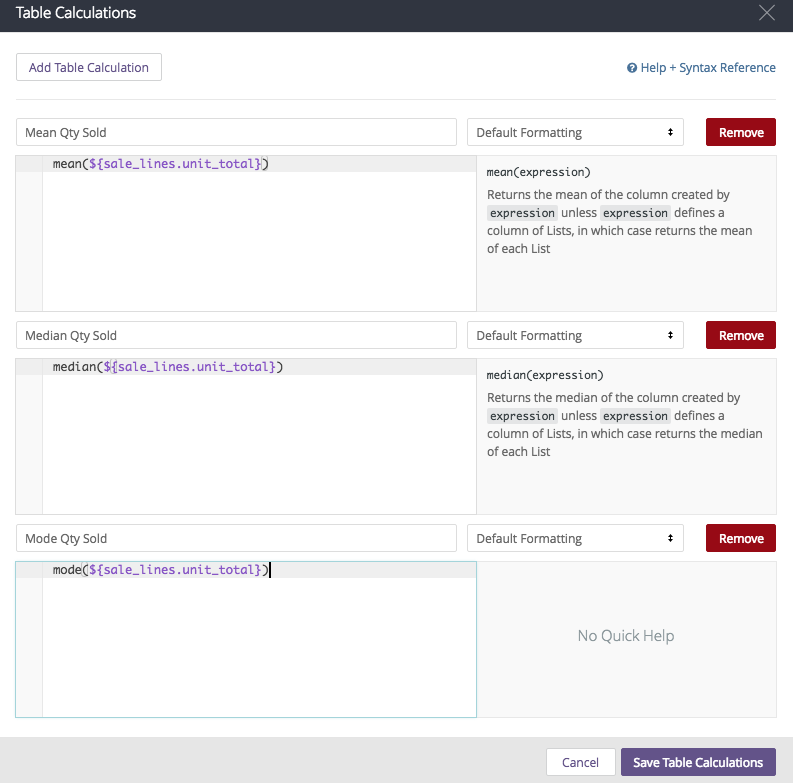In this instance, I am finding the mean, median and mode of the number of items sold on a sale last week

My calculations (respectively) look like:

mean(\${sale_lines.unit_total})
median(\${sale_lines.unit_total})
mode(\${sale_lines.unit_total})

and if I have saved my calculation properly...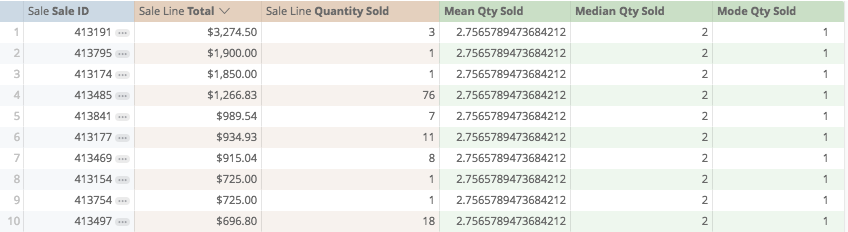# Min

Returns the smallest value from a defined range. Required one argument: defined numeric range.

# Round

Rounds a number up or down to defined number of decimal places.

Say I wanted to look at my total sales for the past six weeks, and determine what the average weekly qty sold is.

Before rounding, my weekly average looks like...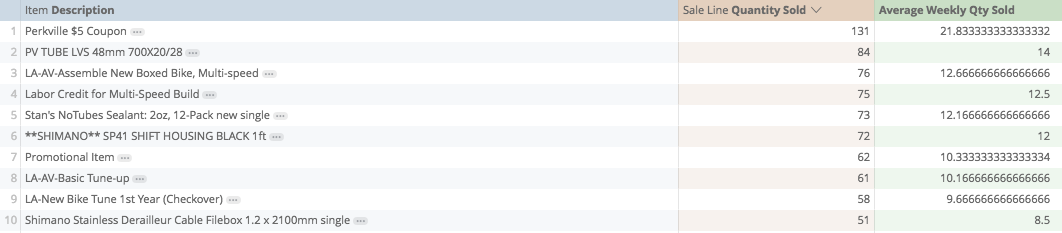The "Round" function needs two arguments, the first argument is which number is being rounded, the second argument is how many decimal places we want to round to: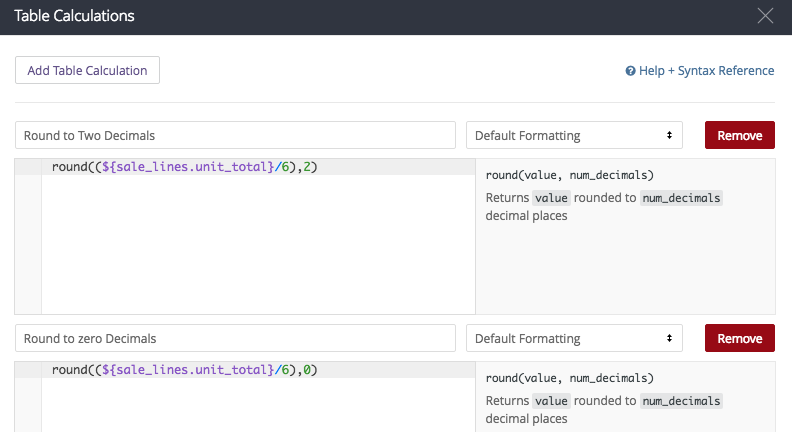So, in this example, the number we want to round is the six-week weekly average: (\${sale_lines.unit_total}/6), and the number of decimals is 2 in the first cell, 0 in the second.

Our calculations look (respectively) like:

round((\${sale_lines.unit_total}/6),2)
round((\${sale_lines.unit_total}/6),0)

and if I have saved my calculations properly...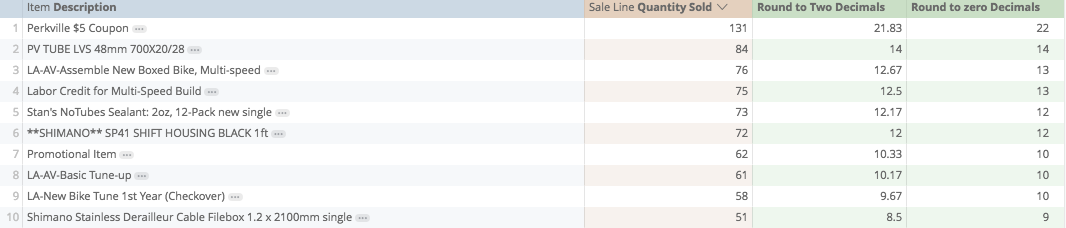# Running Total

Used to add all values in sequence, for example, if I want to see what my year-to-date totals are per day,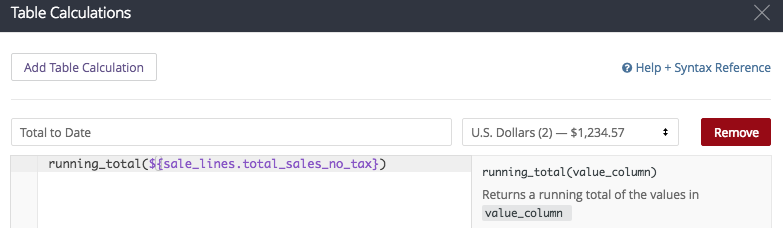In this instance, my calculation looks like:

running_total(\${sale_lines.total_sales_no_tax})

and if I have saved my calculation properly: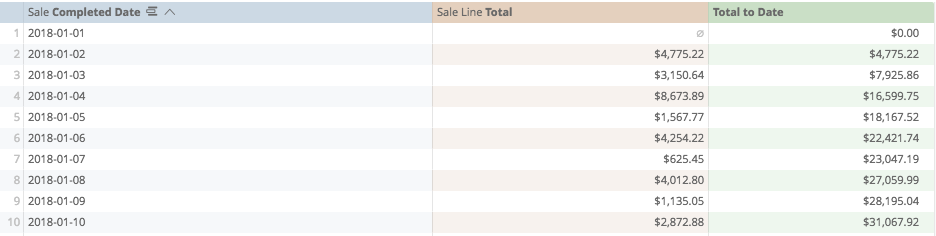# Small

Opposite of "Large", returns the nth smallest value from a range where "n" is freely defined. Requires two arguments, which field to look at, and which smallest number...

For example, if I want to see what my lowest daily sales were...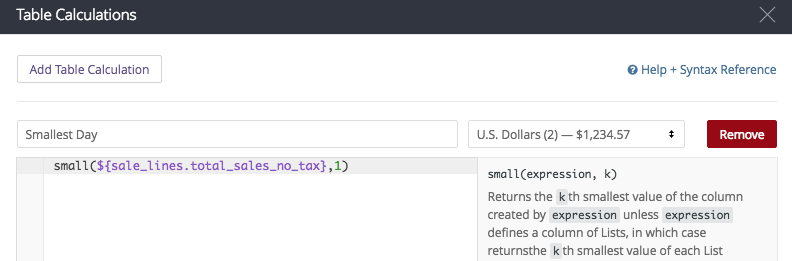my calculation looks like:

small(\${sale_lines.total_sales_no_tax},1)

and if I have saved my calculation properly: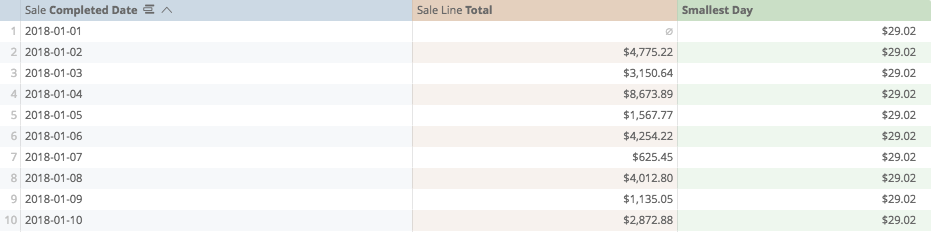# Sum

Returns the total value of a visible range, does not include values not displayed on the table. Requires one argument, which range is being summed.

If I want to return the sum of total sales, for example...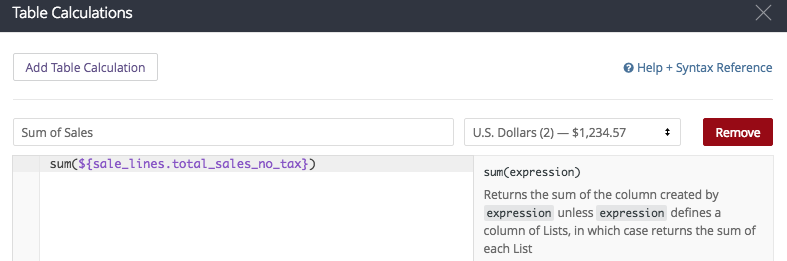in this instance, my calculation looks like,

sum(\${sale_lines.total_sales_no_tax})

and if I have saved it properly...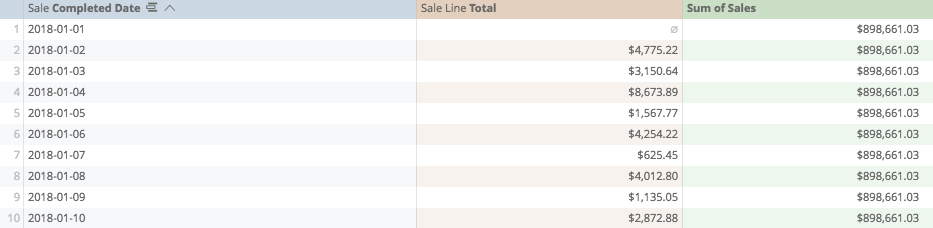Calculations Home
Post edited by josephmckeown on
Joseph McKeown
Lightspeed Analytics and reporting consultant
Lightspeed HQ